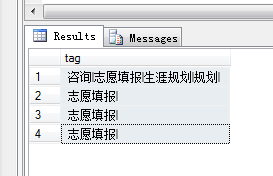###### 我真不懂NET

2015-09-10 10:32 阅读 1.7k

# 字符串分割后重复字符出现频率排序

20• 点赞
• 写回答
• 关注问题
• 收藏
• 复制链接分享

#### 3条回答默认 最新

• 已采纳
`````` string s = "";
foreach (DataRow Row in ds.Tables.Rows)
s += Row["Tag"].ToString() + "|";
vqr query = s.Split("|").Where(x => x != "")
.GroupBy(x => x)
.OrderByDescending(x => x.Count());
string result = string.Join("|", query.Select(x => x.Key).ToArray());
``````
点赞 1 评论 复制链接分享
• foreach (DataRow Row in ds.Tables.Rows)
{
if (Row["Tag"].ToString() == "")
{
isnull = true;
}
else
{
foreach (var item in Row["Tag"].ToString().Remove(Row["Tag"].ToString().Length - 1, 1).Split('|'))
{
if (!LSTag.Contains(item))
{
LSTag.Add(item);
}
else
{

``````                        }
}
}
}
if (!isnull)
{
for (int i = 1; i <= LSTag.Count; i++)
{
sbTag.Append("<li style=\"margin-top:2px;\"><a herf=\"#\" style=\"color:#ffffff;\">").Append(LSTag[i - 1]).Append("    ").Append("</a><span>X</span></li>");
}
}
``````
点赞 2 评论 复制链接分享
• 给个思路吧。我是搞JAVA的.net的话应该差不多。

1、先统计每个单词的个数，使用map存储。key=字符，value=个数（.net可以用direct)
2、然后遍历map，依次输出最大的即可

伪代码：

`````` List<String> tagList = db.select();

List<String> singleTags = new ArrayList<>();

Map<String,Integer> countMap = new HashMap<>();

for(String tag in tagList){  // 遍历查询结果

String [] tags = tag.split["|"]; // 分割字符

for(String t: tags){  // 遍历分割后的字符数组

Integer count  = coutMap.get(t); // 获取当前字符的个数
if(count == null ){  // 不存在则新增，记录个数为1
coutMap.put(t,1)
}else{  // 否则个数+1
coutMap.put(t,++count)
}

}

}

//遍历map.

for key,value in countMap:

// 比较value,最大的就输出key,直到最后一个。 到这一步处理方式就比较多了，可以冒泡，快速等

``````
点赞 1 评论 复制链接分享